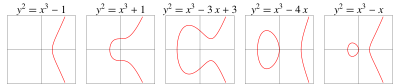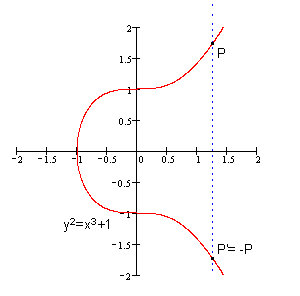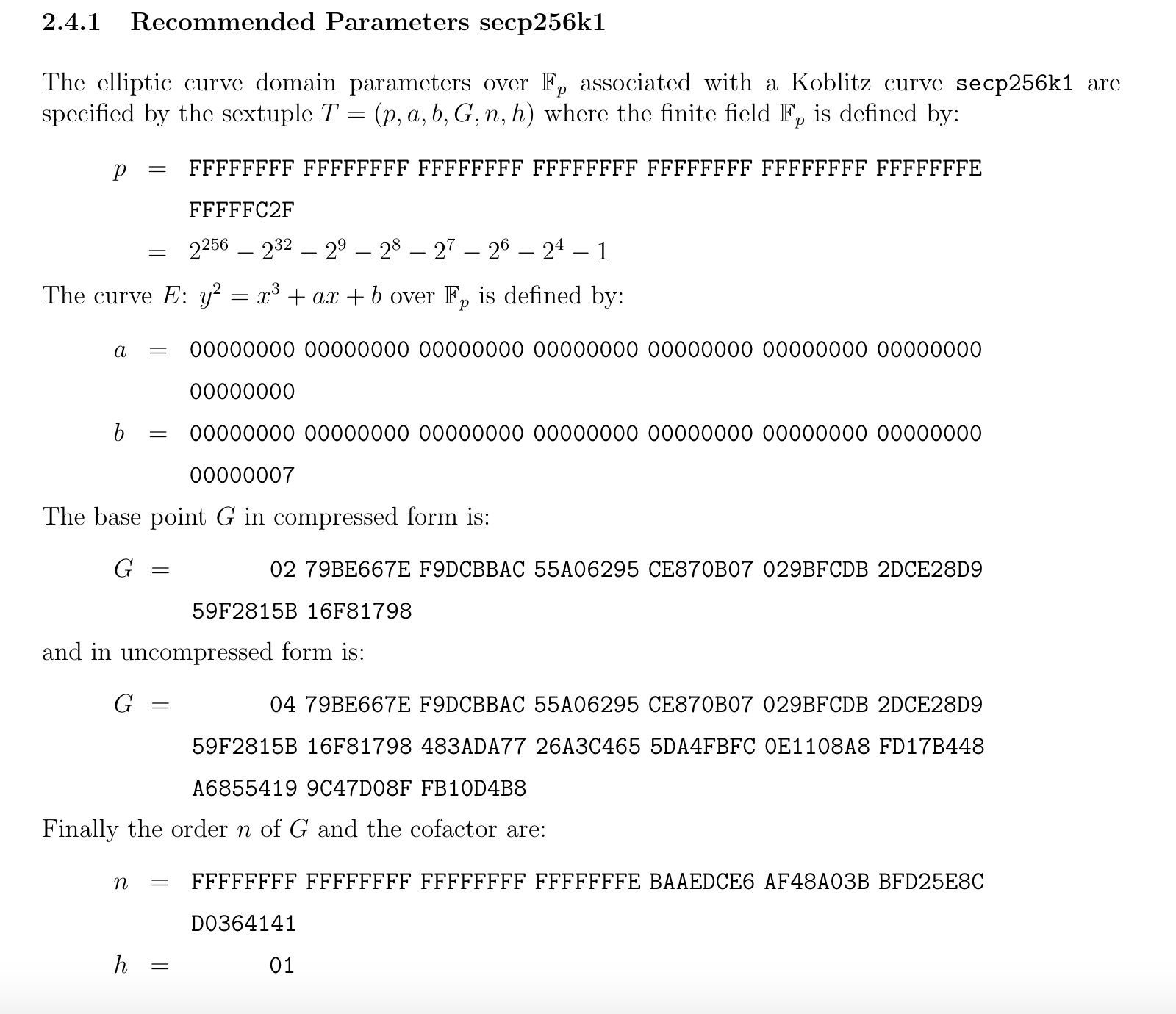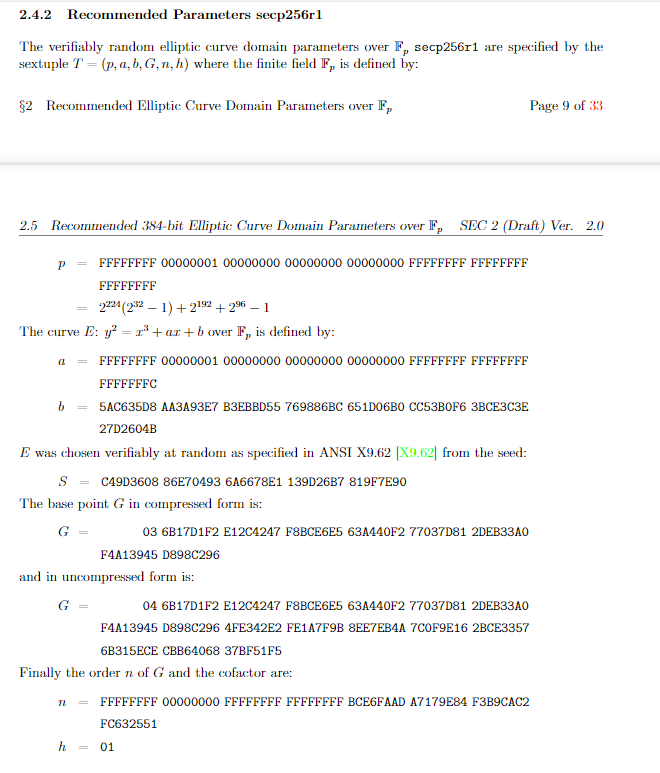# ECC

ECC的主要优势是在某些情况下它比其他的方法使用更小的密钥——比如RSA算法——提供相当的或更高等级的安全。

# 椭圆曲线

$y^2=x^3+ax+b$

$4a^3+27b^2\neq 0$# 椭圆曲线上的阿贝尔群

$$\mathbb{G}$$是一个集合，在集合中定义一个对两个元素的操作，记为"加"，用$$+$$表示，集合中两个元素$$a$$$$b$$，“加”操作表示为$$a+b$$，如果$$a+b$$满足以下5个特性，$$\mathbb{G}$$就是一个阿贝尔群。

• 闭合性（closure）： 若$$a,b$$$$\mathbb{G}$$中的元素，那么$$a+b$$也是。
• 结合性（associativity）： $$(a+b)+c=a+(b+c)$$.
• 存在单位元（identity element 0）：有$$a+0=0+a=a$$.
• 每个元素都有一个相反数，对每个元素$$a$$都存在$$b$$使$$a+b=0$$, $$b$$就是$$a$$的相反数，可以表示为$$−a$$.
• 交换律：$$a+b=b+a$$.

• 一个椭圆曲线上的点是$$\mathbb{G}$$中的元素。
• 单位元$$0$$定义为无穷远处的点。
• 一个点$$P$$的相反数是关于$$x$$轴对称的点。
• “加”定义为：$$P,Q,R$$是一条直线跟椭圆曲线相交的3个点，那么有$$P+Q+R=0$$，也就是$$P+Q=-R$$.# 椭圆曲线的参数

1. 椭圆曲线中的系数$$a,b$$.
2. 有限域的大小是个素数$$p$$.
3. 子群基准点$$G$$.
4. 子群的阶$$n$$.
5. 子群的协因子$$h$$.

## 子群的基准点$$G$$和子群的阶$$n$$

$nG=G+G+\cdots +G$

1. 子群的阶就是子群中的元素个数，等价于$$nG=0$$，其$$n$$是正整数，且$$n$$是其中最小的1个，这个$$n$$就是这个子群的阶。
2. 根据拉格朗日定理，子群的阶，是父群阶的一个因子，比如一个椭圆曲线群有$$N$$个元素，它的一个子群有n个元素，n能整除N.

## 子群的协因子$$h$$

$NP=0$

$n(hP)=0$

# 常用椭圆曲线参数

## secp256k1

secp256k1是高效密码组标准(SECG)协会开发的一套高效的椭圆曲线签名算法标准。在比特币流行之前，secp256k1并未真正使用过。secp256k1命名由几部分组成。

• sec来自SECG标准。
• p表示曲线坐标是素数域。
• 256表示素数是256位长。
• k表示它是Koblitz曲线的变体。
• 1表示它是第一个标准中该类型的曲线。

secp256k1的参数如下。## secp256r1

secp256r1是美国国家安全局建议使用的椭圆曲线，里面的r代表曲线的参数是经过随机选取的。它也被称为NIST P-256# ECDSA算法

## 生成密钥对（genKey）

1. 确定子群的阶数$$n$$，基点$$G$$.
2. 选择随机数$$d\in [1,n)$$作为私钥，并计算出公钥$$Q=d⋅G$$.

## 加密（encrypt）

1. 准备要加密的数据$$M$$，随机数$$r$$.
2. 计算密文：$$C_1=M+r⋅Q,C_2=r⋅G$$

## 解密（decrypt）

1. $$C_1-d⋅C_2=M+r⋅Q-r(d⋅G)=M+r⋅Q-r⋅Q=M$$

## 签名（sign）

1. 对消息$$m$$使用消息摘要算法，得到$$z=hash(m)$$.
2. 生成随机数$$k\in [1,n)$$，计算点$$(x, y)=k⋅G$$.
3. $$r=x\,mod\,n$$，若$$r=0$$则重新选择随机数$$k$$.
4. 计算$$s=k^{−1}(z+rd)\,mod\,n$$，若$$s=0$$则重新选择随机数$$k$$.
5. 上述$$(r,s)$$即为ECDSA签名。

## 验证（verify）

1. 验证$$r,s\in [1,n)$$.
2. 计算$$z=hash(m)$$.
3. 计算$$u_1=zs^{−1}\,mod\,n$$$$u_2=rs^{−1}\,mod\,n$$.
4. 计算$$(x, y)=u_1⋅G+u_2⋅Q\,mod\,n$$.
5. 判断$$r==x$$，若相等则签名验证成功。

## 恢复（recover）

1. 验证$$r, s\in [1,n)$$
2. 计算$$R=(x,y)$$，其中$$x=r,r+n,r+2n...$$，代入椭圆曲线方程计算获得$$R$$.
3. 计算$$z=hash(m)$$.
4. 计算$$u_1=−zr^{−1}\,mod\,n$$$$u_2=sr^{−1}\,mod\,n$$.
5. 计算公钥$$Q= (x’,y’)=u_1⋅G+u_2⋅R$$

# 参考资料

posted @ 2022-03-10 21:04  HachikoT  阅读(126)  评论(0编辑  收藏  举报